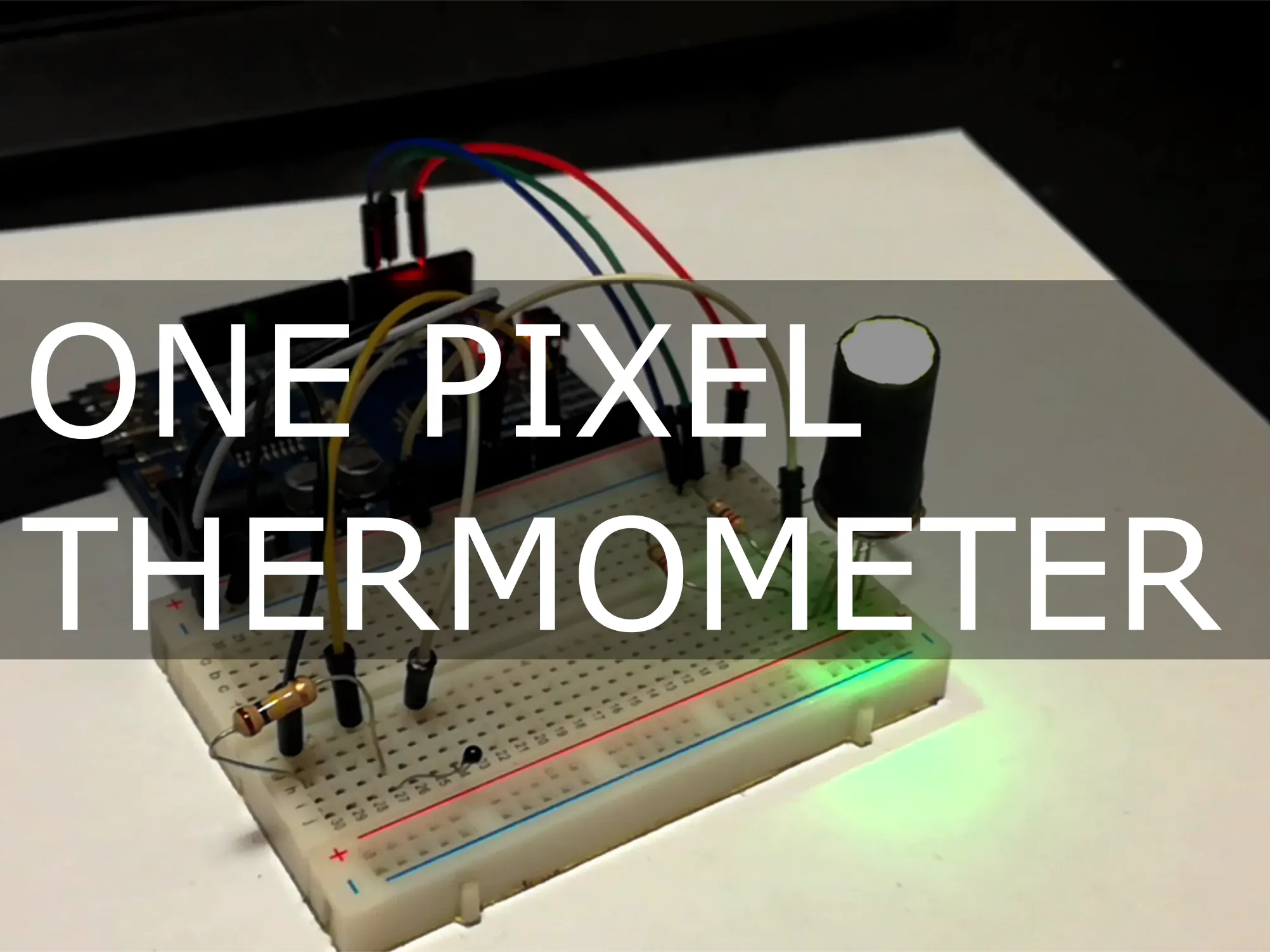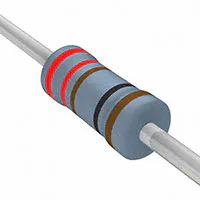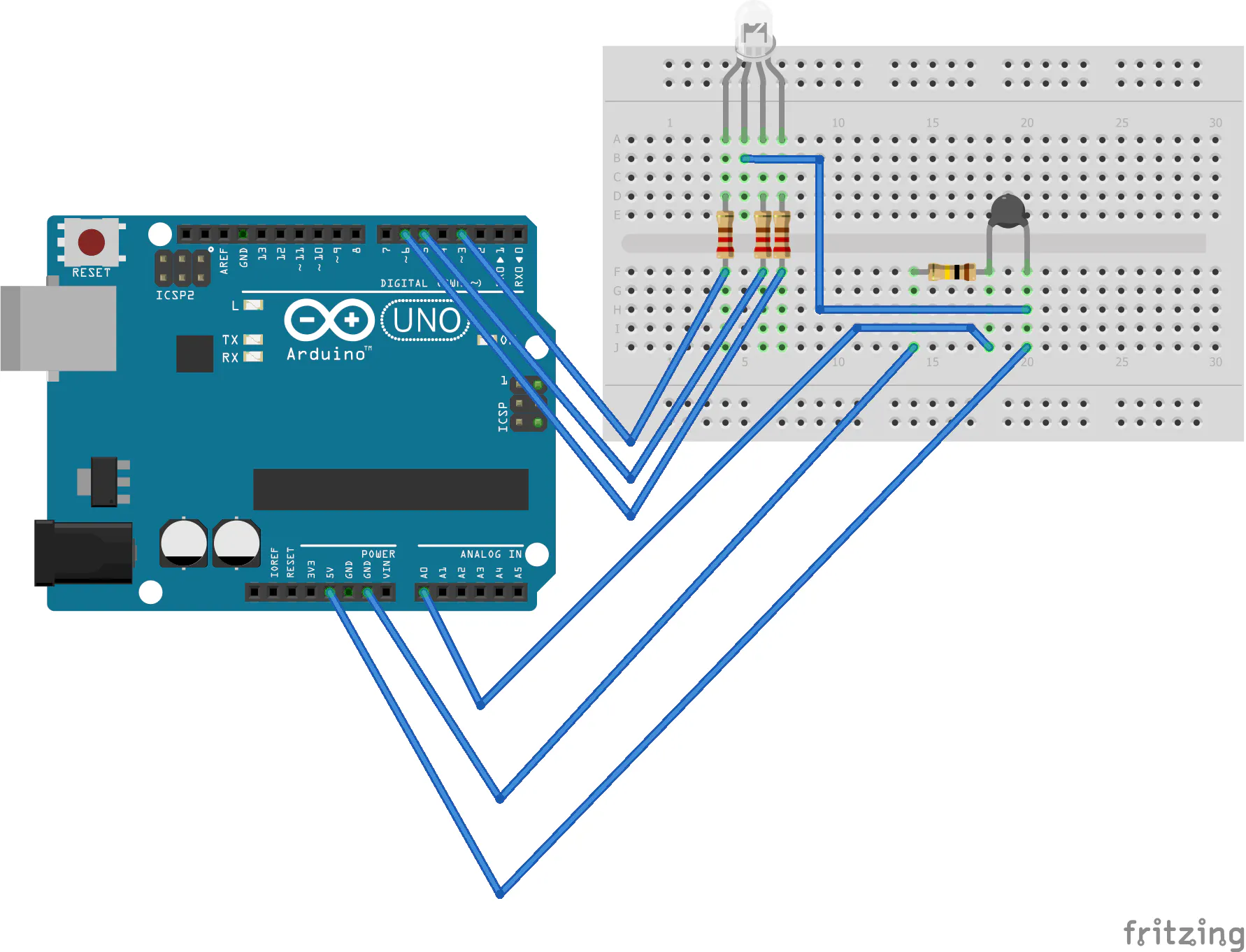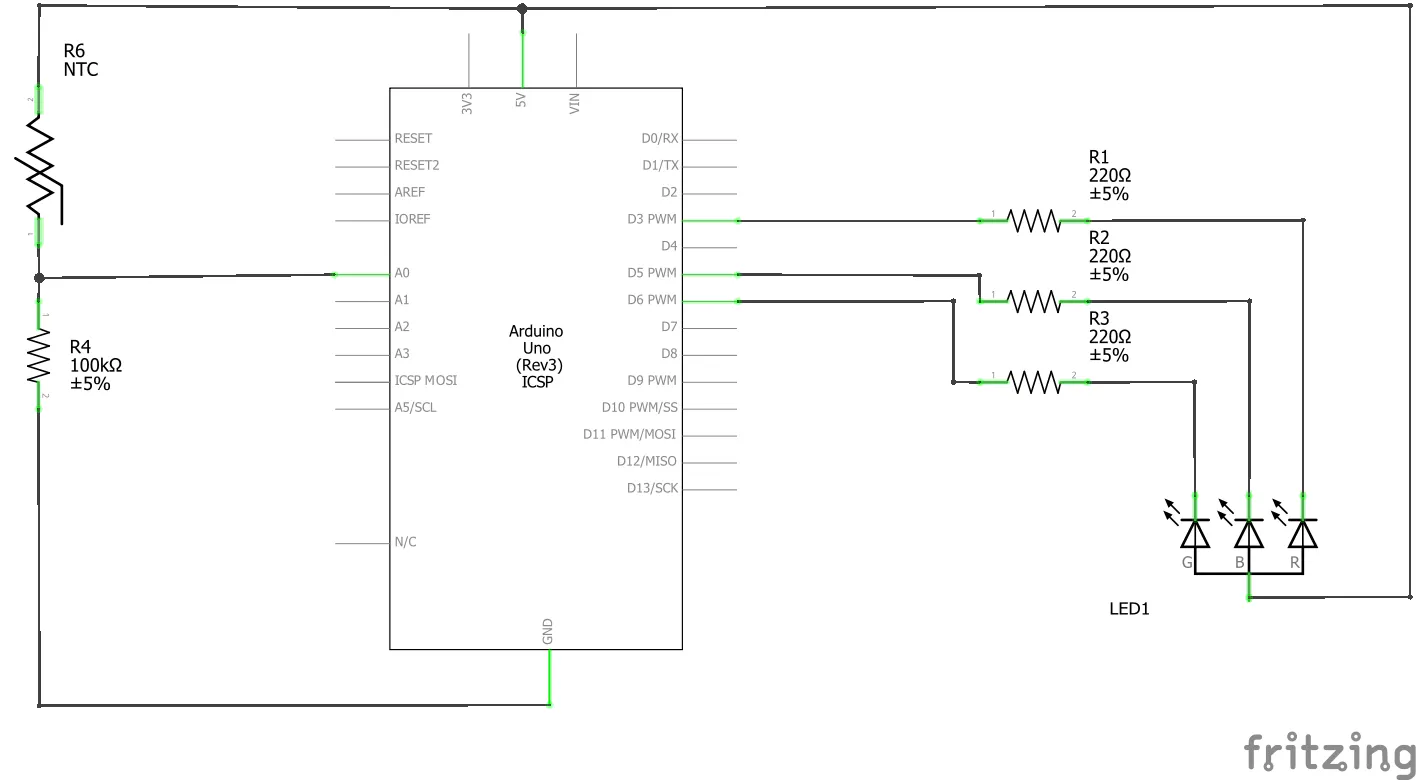# One Pixel Thermometer

Makes use of a thermistor to display a colour on an RGB LED (One Pixel).

BeginnerFull instructions provided1 hour2,989## Things used in this project

### Hardware componentsArduino UNO & Genuino UNO
×1×1Resistor 221 ohm
×3Resistor 10k ohm Should be 100k Ohm Resistor
×1
 RGB LED (Common Anode)
×1
 NTK100 Thermistor
×1

## Schematics### One Pixel Thermometer Schematic

One Pixel Thermometer Schematic## Code

### One Pixel Thermometer

Arduino
Use this code to upload onto your arduino for the One Pixel Thermometer
```/*
One Pixel Thermometer
Controling a RGB LED to indicate temperature
By Ian Cumming https://www.hackster.io/ianabcumming/

H/W: RGB LED, NTK100, 220OHM resistors, 100KOHM Resistor, Arduino UNO.

*/

// ARDUINO UNO PINS

#define minTemp 0
#define maxTemp 42
#define pinR 4     // Red PWM Pin       use 0 on ATTINY85 (pin 5)
#define pinG 0     // Green PWM Pin     use 1 on ATTINY85 (pin 6)
#define pinB 1     // Blue PWM Pin      use 4 on ATTINY85 (pin 3)
#define pinT A1    // Thermistor Pin    use A1 on ATTINY85 (pin 7)

// Variables to calculate Centigrade from the Thermistor Pin input value
int Vo;
float R1 = 10000, logR2, R2, T, c1 = 1.009249522e-03,
c2 = 2.378405444e-04, c3 = 2.019202697e-07; // <<<---- Magic numbers aparently

void setup() {
// Init Serial
//  Serial.begin(115200);

// Init Pins
pinMode(pinR, OUTPUT);
pinMode(pinG, OUTPUT);
pinMode(pinB, OUTPUT);

//Dont really need to do this for the UNO but I'm doing it anyway
pinMode(pinT, INPUT);

}

void loop() {

// Calculate Centigrade : Got function from http://www.circuitbasics.com/arduino-thermistor-temperature-sensor-tutorial/
R2 = R1 * (1023.0 / (float)Vo - 1.0);
logR2 = log(R2);
T = (1.0 / (c1 + c2*logR2 + c3*logR2*logR2*logR2));
T = T - 273.15;

// Map to the range outlined in minTemp and maxTemp
int temp = map(T,minTemp,maxTemp,0,512);

// Assign a RGB value to the range 0 to 512 found in temp
int r = constrain(temp-256,0,255);
int g = constrain(256-abs(temp-256),0,255);
int b = constrain(256-temp,0,255);

// PWM Write to get "faded colours"
// Remove "255 -" if LED's are common Cathode
analogWrite(pinR,255 - r);
analogWrite(pinG,255 - g);
analogWrite(pinB,255 - b);

// Print Temperature to Serial
//  Serial.println(T);
delay(100);
}
```

## Credits

### Ian Cumming

6 projects • 48 followers
I like stuff, from building rockets to elctronics.